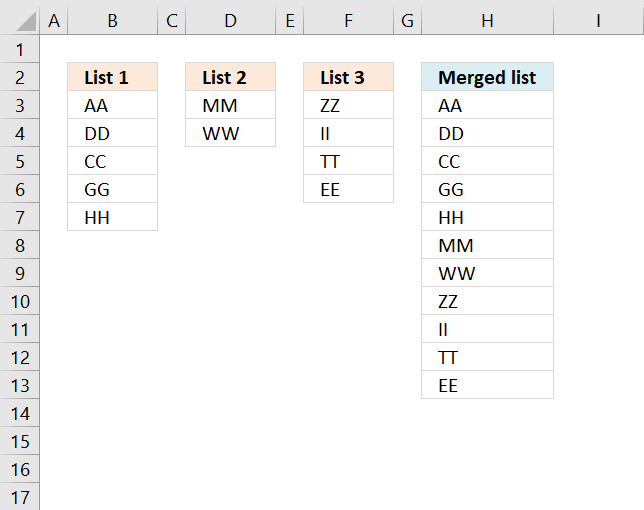Author: Oscar Cronquist Article last updated on September 12, 2022The above image demonstrates a formula that adds values in three different columns into one column.

## 1. Merge three columns into one list - Excel 365Excel 365 subscribers can access new array manipulation formulas that make working with arrays and cell ranges much easier, one of those new functions is the VSTACK function.

It allows you to merge multiple cell ranges vertically, meaning the second cell range/array is joined below the first cell range/array. The result is a dynamic array formula that spills values below as far as needed.

This example shows how to merge three nonadjacent cell ranges with different sizes, cell range B3:B7 has five values. Cell range D3:D4 has two values, and  F3:F6 has four values. The formula is entered in cell H3 and the array values are spilled to cell H3 and cells below as far as needed.

Excel 365 dynamic array formula in cell H3:

=VSTACK(B3:B7, D3:D4, F3:F6)

### Explaining formula

The VSTACK function combines cell ranges or arrays. Joins data to the first blank cell at the bottom of a cell range or array (vertical stacking)

Function syntax: VSTACK(array1,[array2],...)

## 2. Merge three columns into one list - earlier Excel versionsThis example works in earlier Excel versions from 2007 to Excel 2019, it requires a more complicated formula because these versions don't have the VSTACK function.

The IFERROR function is used a lot in this example and I want to warn you that it also handles all formula errors, this may

Formula in H3:

=IFERROR(INDEX(\$B\$3:\$B\$7, ROWS(H2:\$H\$2)), IFERROR(INDEX(\$D\$3:\$D\$4, ROWS(H2:\$H\$2)-ROWS(\$B\$3:\$B\$7)), IFERROR(INDEX(\$F\$3:\$F\$6, ROWS(H2:\$H\$2)-ROWS(\$B\$3:\$B\$7)-ROWS(\$D\$3:\$D\$4)), "")))

Copy cell H2 and paste to the cells below.

### Explaining formula in cell H2

The IFERROR function moves the calculation to the next part (formula2) when the first part (formula1) begins to return errors. That is also true for the second part (formula2), when errors occur the calculation continues with the third part (formula3)

IFERROR(IFERROR(formula1, formula2), formula3)

Formula1 extracts values from List1. Formula2 extracts values from List2. Formula3 extracts values from List3.

#### Step 1 - Count cells vertically

The ROWS function counts rows in a cell reference. H2:\$H\$2 is special, it expands as the formula is copied to the cells below.

ROWS(H2:\$H\$2)

returns 1.

#### Step 2 - Return value

The INDEX function returns values from a cell range based on a row number and column number.

INDEX(\$B\$3:\$B\$7, ROWS(H2:\$H\$2))

becomes

INDEX(\$B\$3:\$B\$7, 1)

becomes

INDEX({"AA";"DD";"CC";"GG";"HH"}, 1)

and returns "AA" in cell H3.

#### Step 3 - Loop

When the formula starts returning errors the second part of the formula begins.

INDEX(\$D\$3:\$D\$4, ROWS(H2:\$H\$2)-ROWS(\$B\$3:\$B\$7))

It also takes into account the number of values returned from the first cell range, for example in cell H8:

INDEX(\$D\$3:\$D\$4, ROWS(H7:\$H\$2)-ROWS(\$B\$3:\$B\$7))

becomes

INDEX(\$D\$3:\$D\$4, 6-ROWS(\$B\$3:\$B\$7))

becomes

INDEX(\$D\$3:\$D\$4, 6-5)

becomes

INDEX({"MM";"WW"}, 1)

and returns "MM" in cell H8.

## 3. Merge three columns into one list - Excel 2003 and earlier versionsFormula in cell H3:

=INDEX((List1, List2, List3), ROW(A1)*(ROW(A1)<=ROWS(List1))+(ROW(A1)-ROWS(List1))*(ROW(A1)>ROWS(List1))*(ROW(A1)<=(ROWS(List1)+ROWS(List2)))+(ROW(A1)-ROWS(List1)-ROWS(List2))*(ROW(A1)>ROWS(List1))*(ROW(A1)>(ROWS(List1)+ROWS(List2)))*(ROW(A1)<=(ROWS(List1)+ROWS(List2)+ROWS(List3))), , (ROW(A1)<=ROWS(List1))*1+(ROW(A1)>ROWS(List1))*(ROW(A1)<=(ROWS(List1)+ROWS(List2)))*2+(ROW(A1)>ROWS(List1))*(ROW(A1)>(ROWS(List1)+ROWS(List2)))*(ROW(A1)<=(ROWS(List1)+ROWS(List2)+ROWS(List3)))*3) + ENTER

Named ranges
List1 (A2:A6)
List2 (B2:B3)
List3 (C2:C5)

## 4. Get Excel file

merge-three-columns.xlsx

merge-three-columns_excel_2003.xls